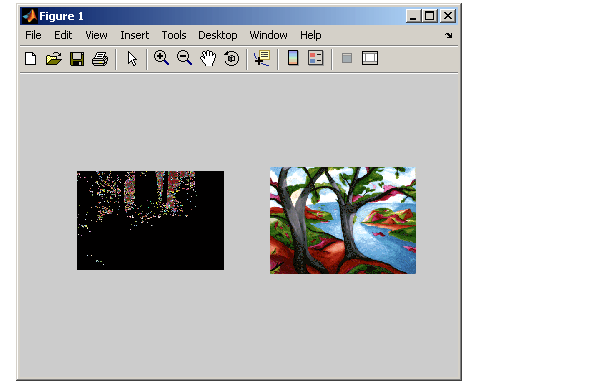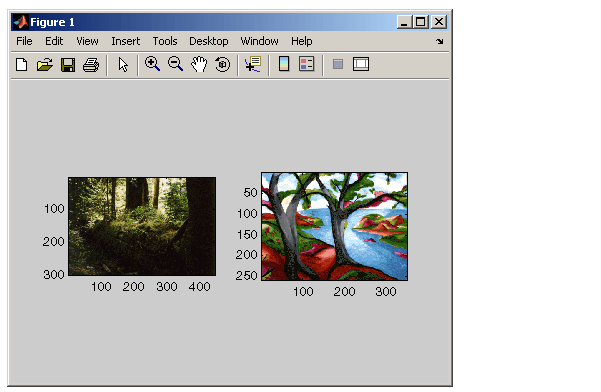Image Processing Toolbox User's GuideDisplaying Multiple Images in the Same Figure

You can use the `imshow` function with the MATLAB `subplot` function or the MATLAB `subimage` function to display multiple images in a single figure window.

 Note    `imtool` does not support this capability.

Dividing a Figure Window into Multiple Display Regions.   `subplot` divides a figure into multiple display regions. The syntax of `subplot` is

• ```subplot(m,n,p)
```

This syntax divides the figure into an `m`-by-`n` matrix of display regions and makes the `p`th display region active.

 Note    When you use `subplot` to display multiple color images in one figure window, the images must share the colormap of the last image displayed. In some cases, as illustrated by the following example, the display results can be unacceptable. As an alternative, you can use the `subimage` function, described in Using the subimage Function to Display Multiple Images, or you can map all images to the same colormap as you load them.

For example, you can use this syntax to display two images side by side.

• ```[X1,map1]=imread('forest.tif');
subplot(1,2,1), imshow(X1,map1)
subplot(1,2,2), imshow(X2,map2)
```

In the figure, note how the first image displayed, `X1`, appears dark after the second image is displayed.Two Images in Same Figure Using the Same Colormap

Using the subimage Function to Display Multiple Images.   `subimage` converts images to truecolor before displaying them and therefore circumvents the colormap sharing problem. This example uses `subimage` to display the forest and the trees images with better results.

• ```[X1,map1]=imread('forest.tif');Displaying Each Image in a Separate Figure Displaying Different Image Types